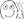# [UDEMY] Machine Learning for Beginners-Regression Analysis in Python

#### Kangaroo69

##### Lurker
Member
Feedback: 0 / 0 / 0
What you'll learn:
• Learn how to solve real life problem using the Linear Regression technique​
• Preliminary analysis of data using Univariate and Bivariate analysis before running Linear regression​
• Predict future outcomes basis past data by implementing Simplest Machine Learning algorithm​
• Understand how to interpret the result of Linear Regression model and translate them into actionable insight​
• Understanding of basics of statistics and concepts of Machine Learning​
• Indepth knowledge of data collection and data preprocessing for Machine Learning Linear Regression problem​
• Learn advanced variations of OLS method of Linear Regression​
• Course contains a end-to-end DIY project to implement your learnings from the lectures​
• How to convert business problem into a Machine learning Linear Regression problem​
• Basic statistics using Numpy library in Python​
• Data representation using Seaborn library in Python​
• Linear Regression technique of Machine Learning using Scikit Learn and Statsmodel libraries of Python​